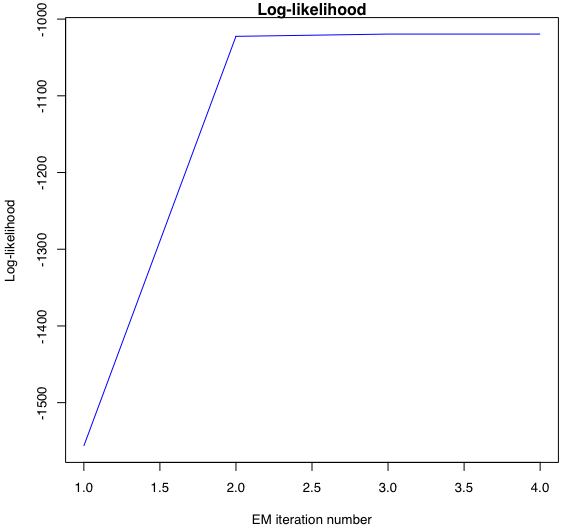# Introduction

HMMR: Flexible and user-friendly probabilistic segmentation of time series (or structured longitudinal data) with regime changes by a regression model governed by a hidden Markov process, fitted by the EM (Baum-Welch) algorithm.

It was written in R Markdown, using the knitr package for production.

See help(package="samurais") for further details and references provided by citation("samurais").

data("univtoydataset")

# Set up HMMR model parameters

K <- 5 # Number of regimes (states)
p <- 3 # Dimension of beta (order of the polynomial regressors)
variance_type <- "heteroskedastic" # "heteroskedastic" or "homoskedastic" model

# Set up EM parameters

n_tries <- 1
max_iter <- 1500
threshold <- 1e-6
verbose <- TRUE

# Estimation

hmmr <- emHMMR(univtoydataset$x, univtoydataset$y, K, p, variance_type, n_tries,
max_iter, threshold, verbose)
## EM: Iteration : 1 || log-likelihood : -1556.39696825601
## EM: Iteration : 2 || log-likelihood : -1022.47935723687
## EM: Iteration : 3 || log-likelihood : -1019.51830707432
## EM: Iteration : 4 || log-likelihood : -1019.51780361388

# Summary

hmmr$summary() ## --------------------- ## Fitted HMMR model ## --------------------- ## ## HMMR model with K = 5 components: ## ## log-likelihood nu AIC BIC ## -1019.518 49 -1068.518 -1178.946 ## ## Clustering table (Number of observations in each regimes): ## ## 1 2 3 4 5 ## 100 120 200 100 150 ## ## Regression coefficients: ## ## Beta(K = 1) Beta(K = 2) Beta(K = 3) Beta(K = 4) Beta(K = 5) ## 1 6.031872e-02 -5.326689 -2.65064 120.8612 3.858683 ## X^1 -7.424715e+00 157.189455 43.13601 -474.9870 13.757279 ## X^2 2.931651e+02 -643.706204 -92.68115 598.3726 -34.384734 ## X^3 -1.823559e+03 855.171715 66.18499 -244.5175 20.632196 ## ## Variances: ## ## Sigma2(K = 1) Sigma2(K = 2) Sigma2(K = 3) Sigma2(K = 4) Sigma2(K = 5) ## 1.220624 1.111487 1.080043 0.9779724 1.028399 # Plots ## Predicted time series and predicted regime probabilities hmmr$plot(what = "predicted")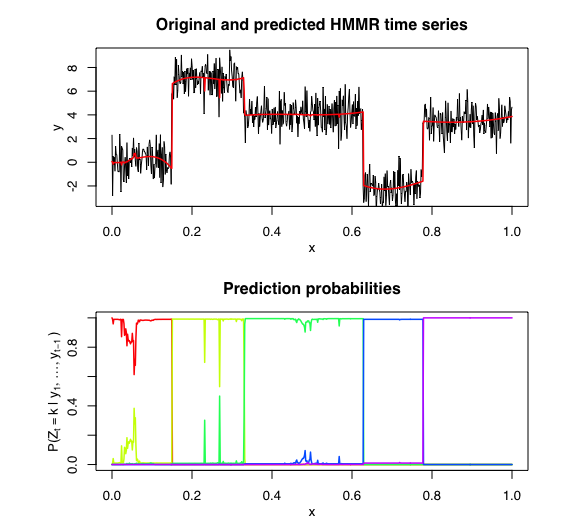hmmr$plot(what = "filtered")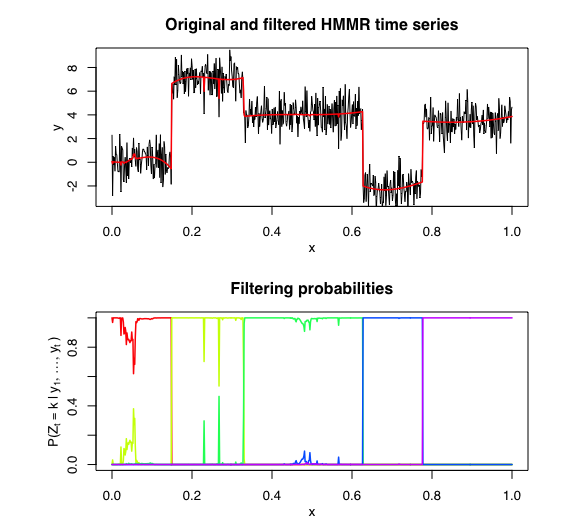## Fitted regressors hmmr$plot(what = "regressors")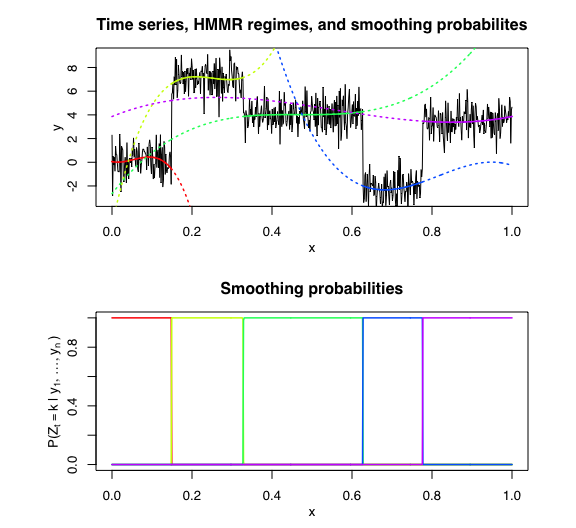hmmr$plot(what = "smoothed")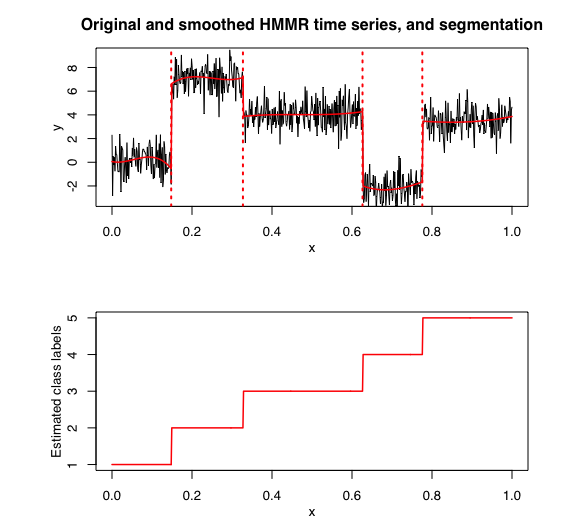## Log-likelihood hmmr$plot(what = "loglikelihood")